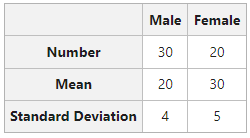# Combined Standard Deviation: Meaning, Formula, and Example

A scientific measure of dispersion, which is widely used in statistical analysis of a given set of data is known as Standard Deviation. Another name for standard deviation is Root Mean Square Deviation. Standard Deviation is denoted by a Greek Symbol σ (sigma). Under this method, the deviation of values is taken from the arithmetic mean of the given set of data.

According to Spiegel, “The Standard Deviation is the square root of the arithmetic mean of the squares of all deviations. Deviations being measured from arithmetic mean of the items.”

Standard Deviation is considered to be the best way of determining the dispersion of a data set. It is because standard deviation takes into account every value of a data set along with its algebraic signs.

### What is Combined Standard Deviation?

Calculating standard deviation of two or more series is known as Combined Standard Deviation. The formula of Combined Standard Deviation in the case of two series is:Where,= Combined Standard Deviation of two groups=Standard Deviation of first group= Standard Deviation of second group= Combined Arithmetic Mean of two groups= Arithmetic Mean of first group= Arithmetic Mean of second group= Number of Observations in the first group= Number of Observations in the second group

The formula of Combined Standard Deviation can be extended up to N number of series. For example, if there are three series, then the formula for Combined Standard Deviation will be:Where,#### Example:

With the help of the following information, calculate Combined Standard Deviation.#### Solution:

First of all, we have to calculate Combined Mean of the given two series.In the given question,Combined Standard Deviation:Combined Standard Deviation = 6.6

Whether you're preparing for your first job interview or aiming to upskill in this ever-evolving tech landscape, GeeksforGeeks Courses are your key to success. We provide top-quality content at affordable prices, all geared towards accelerating your growth in a time-bound manner. Join the millions we've already empowered, and we're here to do the same for you. Don't miss out - check it out now!

Previous
Next